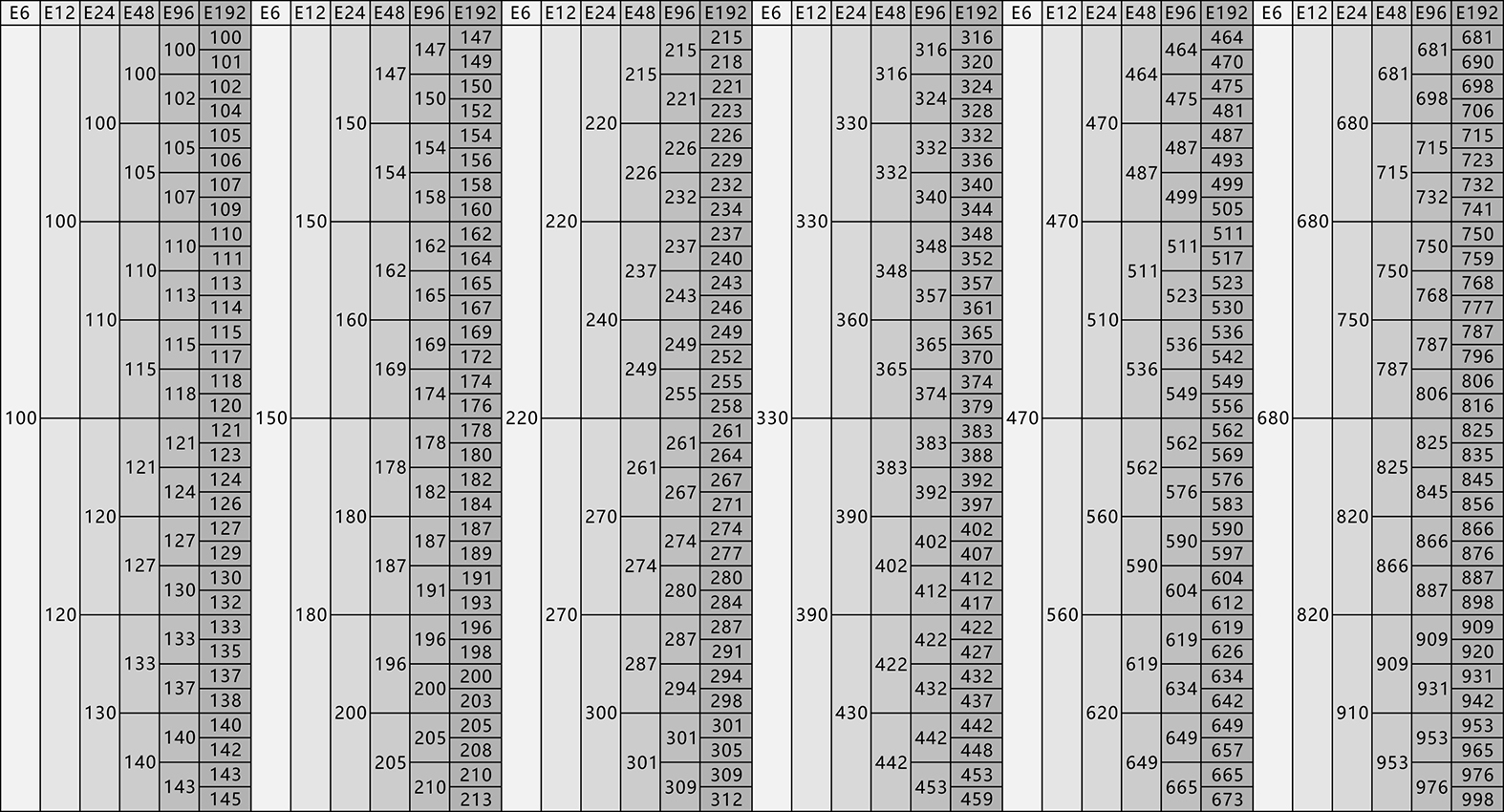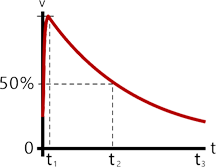• μV
• mV
• V
• KV

• μΩ
• Ω

• pA
• nA
• μA
• mA
• A

• W
• KW

• μΩ
• Ω
• μΩ
• Ω

• pF
• nF
• μF
• mF
• F
• pF
• nF
• μF
• mF
• F

• nH
• μH
• mH
• H
• nH
• μH
• mH
• H（100Ω≤R≤1000Ω）

能量脉冲E=脉冲能量
C=电容
Vpeak=峰值电压
E=½CV²
• pF
• nF
• μF
• mF
• F
* 电容
• μV
• mV
• V
• KV
* 峰值电压

平均功率• ns
• μs
• ms
• s
* 周期
• W
• KW

• J

正常功率Vpeak=峰值电压
t1=上升时间
t2=降低至Vpeak/2时间
t3=总衰减时间
• μV
• mV
• V
• KV
* 峰值电压
• μΩ
• Ω
* 阻值
• ns
• μs
• ms
• s
* t1
• ns
• μs
• ms
• s
* t2
• ns
• μs
• ms
• s
t3

平均功率• ns
• μs
• ms
• s
* 周期
• W
• KW

• J

方波脉冲E=脉冲能量
p=脉冲功率
t=脉冲持续时间
E=pt
• W
• KW

• ns
• μs
• ms
• s
* 脉宽

• μV
• mV
• V
• KV

• pA
• nA
• μA
• mA
• A

• μΩ
• Ω

平均功率• ns
• μs
• ms
• s
* 周期
• W
• KW

• J

资讯中心

2021/09/16
2021/09/08
2021/06/02
2020/06/01
2021-11-18 18:53:24

2021-09-29 11:55:33

2021-08-04 19:31:14

2021-07-28 19:39:12

2021-07-21 18:40:16

2021-07-07 18:34:20